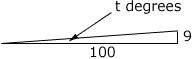Quandaries and Queries dWho is asking: Parent Level: Secondary Question:Ω The gradiant of a hill is 9%. What angle is created by the run/rise of the hill and 0 degrees? Hi Jim, If the gradient is 9% = 9/100 then the rise over the run is 9 over 100.If t is the angle created by the hill and 0 degrees as in the diagram then tan(t) = 9/100 = 0.09 Hence t = tan-1(0.09) Penny Go to Math Central